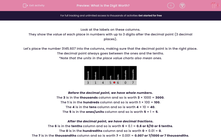# What is the Digit Worth?

In this worksheet, students must identify the value of each digit in numbers given to three decimal places.This content is premium and exclusive to EdPlace subscribers.Key stage:  KS 2

Curriculum topic:   Maths and Numerical Reasoning

Curriculum subtopic:   Place Value

Difficulty level:#### Worksheet Overview

Look at the labels on these columns.

They show the value of each place in numbers with up to 3 digits after the decimal point (3 decimal places).

Let's place the number 3145.607 into the columns, making sure that the decimal point is in the right place.

The decimal point always goes between the ones and the tenths.

*Note that the units in the place value charts also mean ones.Before the decimal point, we have whole numbers.

The 3 is in the thousands column and so is worth 3 × 1000 = 3000.

The 1 is in the hundreds column and so is worth 1 × 100 = 100.

The 4 is in the tens column and so is worth 4 × 10 = 40.

The 5 is in the ones/units column and so is worth 5 × 1 = 5.

After the decimal point, we have decimal fractions.

The 6 is in the tenths column and so is worth 6 × 0.1 = 0.6 or 6/10 or 6 tenths.

The 0 is in the hundredths column and so is worth 0 × 0.01 = 0.

The 7 is in the thousandths column and so is worth 7 × 0.001 = 0.007 or 7/1000 or 7 thousandths.

Example

What is the 7 worth in the number 12.275 ?